# While Loop in C

## While Loop in C Language with Examples

In this article, I will discuss While Loop in C Language with Definitions, Syntax, Flow Charts, and Examples. Please read our previous articles discussing Switch Statements in C Language with Examples. Loop Control Statements are very, very important for logical programming. The Looping Statements are also called Iteration Statements. So, we can use the words Looping and Iteration, and the meanings are the same.

##### What is Looping?

Repeating a statement or group of statements until the condition is satisfied is called looping. In this case, when the condition becomes false, the execution of the loops terminates. The way it repeats the execution of the statements will form a circle; that’s why iteration statements are called loops.

##### Why do we need Looping?

The basic purpose of the loop is code repetition. Whenever repetitions are required, we need to go for looping instead of writing the statements again and again.

##### Iteration or Looping Statements in C:

A loop is nothing but executes a block of instructions repeatedly as long as the condition is true. How many times it will repeat means as long as the given condition is true. When the condition fails, it will terminate the loop execution. C provides the following loop for iteration statements:

1. while loop
2. for loop
3. do-while loop
##### What is While Loop in C Language:

A while loop executes a statement repeatedly until a given condition returns false. Here, statements may be a single statement or a block of statements. The condition may be any expression, and true is any nonzero value. The loop iterates while the condition is true. If you see the syntax and flow chart parallelly, you will get more clarity of the while loop.

###### While Loop Syntax in C Language:

A while loop in C language is a control flow statement that allows code to be executed repeatedly based on a given boolean condition. Following is the syntax to use the while loop in C Programming Language.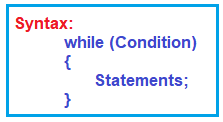Here,

• Condition: The loop starts with the evaluation of a condition. This condition is a boolean expression (i.e., an expression that evaluates to true or false).
• Code Execution: If the condition evaluates to true, the code inside the loop’s curly braces {} is executed.
• Condition Re-evaluation: After the code inside the loop is executed, the condition is evaluated again.
• Loop Continuation: If the condition is still true, the loop executes again. This process repeats until the condition is evaluated to be false.
• Exit Loop: When the condition evaluates to false, the loop is exited, and the program continues with the next line of code after the loop.

It is similar to the if condition, just condition, and statements, but the flow differs from the if condition. How it is different lets us understand it through the flow chart.

##### Flow Chart of While Loop in C Language:

The following diagram shows the flow chart of the while loop.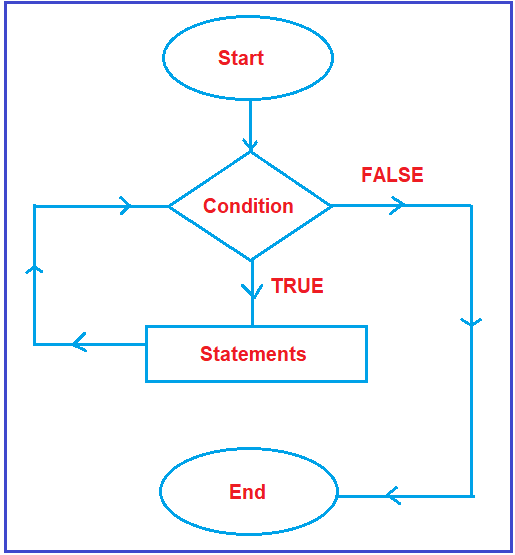The flow chart will start. The oval symbol represents the start. Then, it will check the condition. As discussed earlier, every condition has two outputs, i.e., true and false. If it is true, what will happen, and if it is false, what will happen? We need to check.

Suppose the condition is true; all statements defined inside the block (within the while loop block) will execute. After the execution of statements, will it end? No, it will not end. After the execution of statements, it will go and check the condition once again. It will repeat the same process as long as the given condition is true. Suppose the condition is false, then it will come to an end. This is the execution flow of a while loop.

##### Example: Basic while Loop
```#include <stdio.h>

int main() {
int count = 0;
while (count < 5) {
printf("Count is: %d\n", count);
count++;
}
return 0;
}```

This loop prints numbers from 0 to 4. The loop continues as long as the count is less than 5.

##### Example: Infinite while Loop
```#include <stdio.h>

int main() {
while (1) {
printf("This loop will run forever.\n");
}
return 0;
}```

This is an infinite loop. The condition 1 is always true, so the loop never ends.

##### Example: While Loop with a Break Statement
```#include <stdio.h>

int main() {
int count = 0;
while (count < 10) {
if (count == 5) {
break; // Exit the loop when count reaches 5
}
printf("Count is: %d\n", count);
count++;
}
return 0;
}```

This loop will terminate when the count reaches 5, even though the condition is to loop until the count is less than 10.

##### Example: While Loop with a Continue Statement
```#include <stdio.h>

int main() {
int count = 0;
while (count < 5) {
count++;
if (count == 3) {
continue; // Skip the rest of the loop body for count 3
}
printf("Count is: %d\n", count);
}
return 0;
}```

This loop skips printing the number 3 but continues with the other numbers.

##### Example: Print the nos 10, 9, 8…. 1 using the while loop
```#include <stdio.h>
int main()
{
int i;
i = 10;
while(i >= 1)
{
printf("%d ", i);
i = i - 1;
}
return 0;
}```

Output: 10 9 8 7 6 5 4 3 2 1

Example: Print the numbers in the following format up to a given number, and that number is entered from the keyboard.

2 4 6 8 …………………….. up to that given number

```#include <stdio.h>
int main()
{
int i, n;
printf("Enter a Number : ");
scanf("%d", &n);
i = 2;
while(i <= n)
{
printf("%d ", i);
i = i + 2;
}
return 0;
}```
###### Output: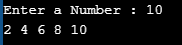###### Program to Enter a number and print the Fibonacci series up to that number using a while loop in C Language.
```#include <stdio.h>
int main ()
{
int i, n, j, k;
printf ("Enter a Number : ");
scanf ("%d", &n);

i = 0;
j = 1;
printf ("%d %d ", i, j);
k = i + j;
while (k <= n)
{
printf (" %d", k);
i = j;
j = k;
k = i + j;
}

return 0;
}```
###### Output: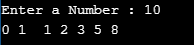Example: Enter a number from the keyboard and then calculate the number of digits and the sum of digits of that number using a while loop.

```#include <stdio.h>
int main()
{
int no, nd, sd, rem;
printf("Enter a Number : ");
scanf("%d", &no);
nd = 0;
sd = 0;
while (no > 0)
{
rem = no % 10;
nd = nd + 1;
sd = sd + rem;
no = no / 10;
}
printf("The number of digit is %d", nd);
printf("\nThe sum of digit is %d", sd);
return 0;
}```
###### Output: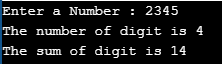##### What is the pre-checking process or entry-controlled loop in C?

The pre-checking process or entry-controlled loop in C refers to loops that evaluate the condition before entering the loop body. The most common examples of entry-controlled loops in C are the while loop and the for loop. In these loops, the condition is checked first, and the loop body is executed only if the condition is true. If the condition is false from the beginning, the loop body does not execute even once.

Key Characteristics of Pre-Checking Loops

• Condition Evaluation at Start: The key characteristic of these loops is that the condition is evaluated before the loop body is executed on each iteration.
• Zero Iterations Possible: Because the condition is checked first, these loops can execute zero times if the condition is false at the outset.
• Safety Against Infinite Loops: While they can still become infinite loops if the condition is always true, the pre-checking nature makes it somewhat safer, as the loop won’t start if the condition is false initially.

### 3 thoughts on “While Loop in C”

1.Read a set of n numbers (n is input) and print if each given number is smaller or bigger than the previous number. For first number there will not be any output as there is no previous number.
eg., input number of values: 5
enter 1st value: 6
enter 2nd value: 7
7 is bigger than 6
enter 3rd value: 5
5 is smaller than 7
enter 4th value: 18
18 is bigger than 5
enter 5th value: 6
6 is smaller than 18

2.#include

int main()
{
int f1,f2,n,i=1;
printf(“Enter n value\n”);
scanf(“%d”,&n);
printf(“enter %d number\n”,i);
scanf(“%d”,&f1);
while(if1)
{
printf(“%d is bigger then%d\n”,f2,f1);
f1=f2;
}
else if (f2<f1)
{
printf("%d is smaller then%d\n",f2,f1);
f1=f2;
}

}

return 0;
}

1.How to work this?